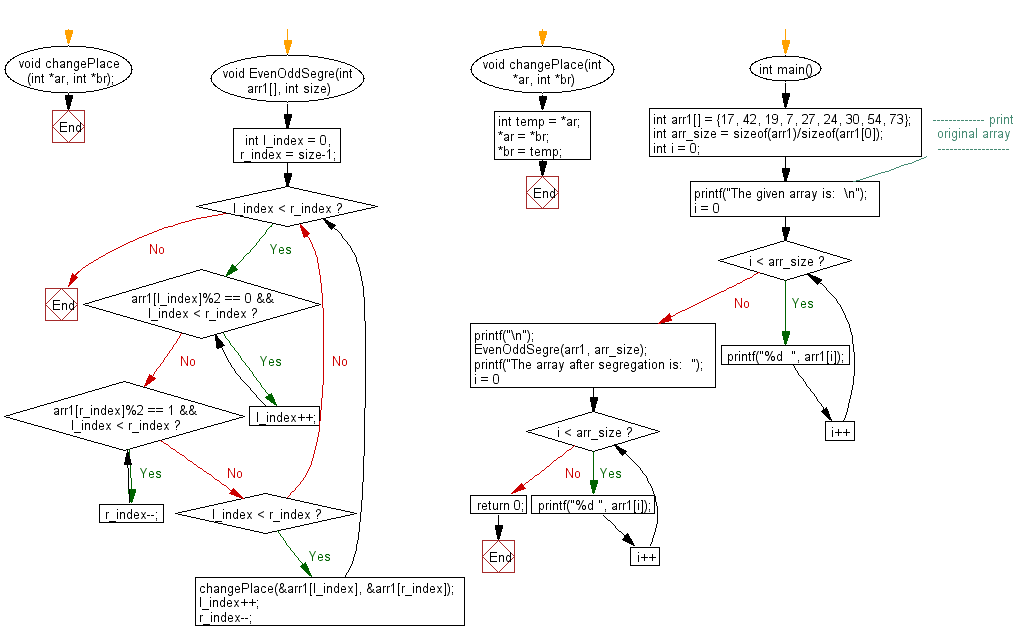﻿ C exercises: Segregate even and odd elements on an array - w3resource# C Exercises: Segregate even and odd elements on an array

## C Array: Exercise-96 with Solution

Write a program in C to segregate even and odd elements on an array.

Sample Solution:

C Code:

``````#include<stdio.h>

void changePlace (int *ar, int *br);
void EvenOddSegre(int arr1[], int size)
{
int l_index = 0, r_index = size-1;
while (l_index < r_index)
{
while (arr1[l_index]%2 == 0 && l_index < r_index)
l_index++;
while (arr1[r_index]%2 == 1 && l_index < r_index)
r_index--;
if (l_index < r_index)
{
changePlace(&arr1[l_index], &arr1[r_index]);
l_index++;
r_index--;
}
}
}

void changePlace(int *ar, int *br)
{
int temp = *ar;
*ar = *br;
*br = temp;
}

int main()
{
int arr1[] = {17, 42, 19, 7, 27, 24, 30, 54, 73};
int arr_size = sizeof(arr1)/sizeof(arr1);
int i = 0;
//------------- print original array ------------------
printf("The given array is:  \n");
for(i = 0; i < arr_size; i++)
{
printf("%d  ", arr1[i]);
}
printf("\n");
//-----------------------------------------------------------
EvenOddSegre(arr1, arr_size);
printf("The array after segregation is:  ");
for (i = 0; i < arr_size; i++)
printf("%d ", arr1[i]);
return 0;
}
```
```

Sample Output:

```The given array is:
17  42  19  7  27  24  30  54  73
The array after segregation is:  54 42 30 24 27 7 19 17 73
```

Pictorial Presentation:Flowchart:C Programming Code Editor:

Improve this sample solution and post your code through Disqus.

﻿

## C Programming: Tips of the Day

Why does c = ++(a+b) give compilation error?

A It's just a rule, that's all, and is possibly there to (1) make it easier to write C compilers and (2) nobody has convinced the C standards committee to relax it.

Informally speaking you can only write ++foo if foo can appear on the left hand side of an assignment expression like foo = bar. Since you can't write a + b = bar, you can't write ++(a + b) either.

There's no real reason why a + b couldn't yield a temporary on which ++ can operate, and the result of that is the value of the expression ++(a + b).

Ref : https://bit.ly/34hP4oT